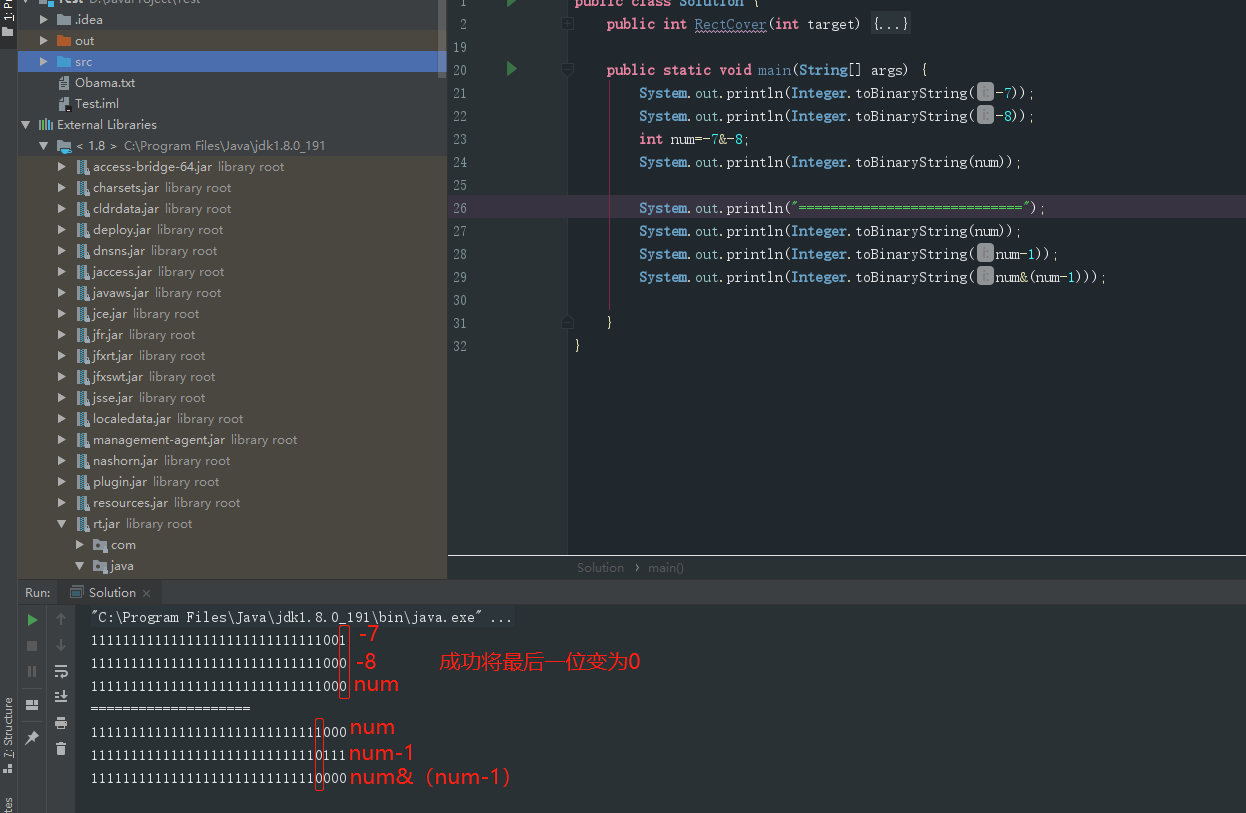• 累计撰写 141 篇文章
• 累计创建 37 个标签
• 累计收到 11 条评论

剑指Offer【11】--二进制中1的个数2020-11-03 / 0 评论 / 0 点赞 / 161 阅读 / 0 字

[toc]

思路以及解法

public class Solution {
public int NumberOf1(int n) {
int num = 0;
while (n != 0) {
int tmp = n % 2;
if (tmp == 1||tmp==-1) ++num;
n /= 2;
}
return num;
}
}

public class Solution {
public int NumberOf1(int n) {
int num=0;
while (n != 0) {
if ((n & 1)==1){
++num;
}
n = n>>1;
}
return num;
}
}

public class Solution {
public int NumberOf1(int n) {
int num = 0, flag = 1;
while (flag != 0) {
if ((n & flag) != 0) {
num++;
}
flag <<= 1;
}
return num;
}
}

32位-7 = 11111111111111111111111111111001 ，
32位-8 = 11111111111111111111111111111000，
-7&-8 = 11111111111111111111111111111000public class Solution {
public int NumberOf1(int n) {
int num = 0;
while (n != 0) {
num++;
n &= (n - 1);
}
return num;
}
}

public class Solution {
public int NumberOf1(int n) {
int t = 0;
String str = Integer.toBinaryString(n);
for (int i = 0; i < str.length(); i++) {
if (str.charAt(i) == '1') {
t++;
}
}
return t;
}
}
0